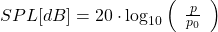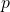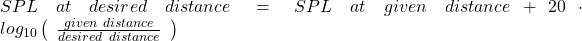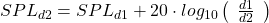Published:

# How do you calculate SPL at one distance to SPL at another distance?

As everyone knows the further away a sound source is, the quieter the sound will be. This is due to the surface expansion of the affected space. One can imagine an ideal point source producing a sound field that moves like an expanding sphere into all 3 dimensions. This means that for doubling the distance the occupied surface gets quadrupled, reducing the energy per m² by a factor of 4.

If we put this into an equation we first must state the equation for the SPL depending on the air pressure:being the actual sound pressure.being the reference sound pressure (20µPa), which is the average hearing threshold at 1kHz of humans.

If one now wants to calculate the SPL at the desired distance from an SPL at a given distance the following equations have to be used:Or in “nice” mathematical writing:Sidenote: This whole chapter is only relevant if the sound is moving like a wave through the air. It does not apply in case of In-ear / artificial ear measurements. There the speaker directly compresses the air between speaker and microphone/eardrum, rendering distance irrelevant as a parameter.

Tags:

Categories: